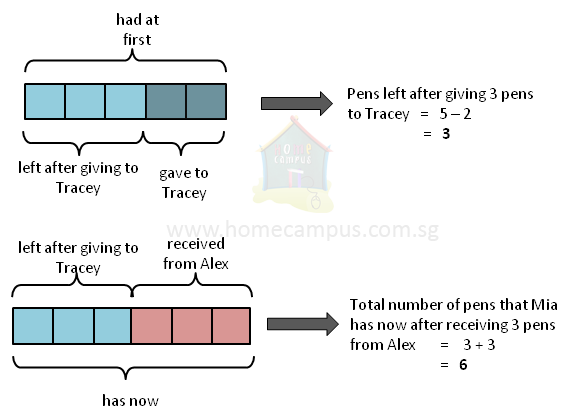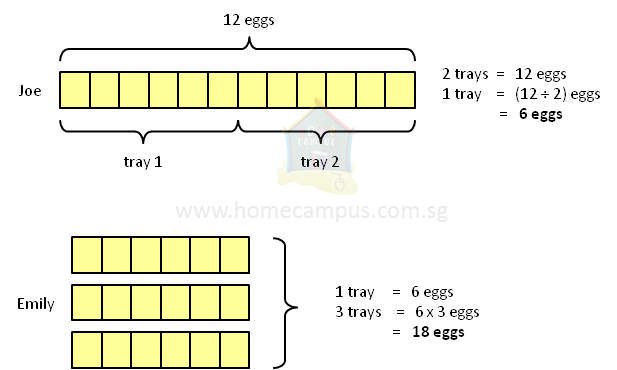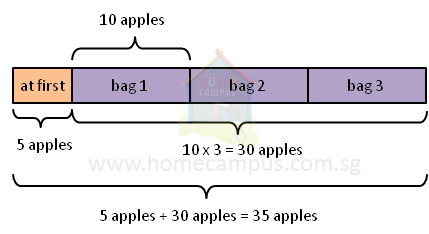## What is Correct Order of Operations?

Practice Unlimited Questions

#### Introduction

The order in which you do operations will determine the answer that you get. So, to get to the correct answer you must follow the right order of operations.
The standard order of operations is:

Step 1: Do the operations within brackets.
Step 2: Do all multiplication and/or division from left to right.
Step 3: Do all addition and/or subtraction from left to right.

#### 1.  Mia had 5 pens. She gave 2 to Tracey and received 3 more from Alex. How many pens does she have now?

The number statement for this problem is:
5  −  2  +  3

Which of the following 2 methods is the correct way to solve this problem?
Method 1: (do subtraction first)
5  −  2  =  3
3  +  3  =  6
Mia has 6 pens now.
2  +  3  =  5
5  −  5  =  0
Mia has no pens now.
Let's draw a model diagram to solve this problem.Mia has 6 pens now.

Therefore, Method 1 is the correct way to solve this problem.

Rule:
Do all addition and/or subtraction from left to right.

#### 2. Joe divided 12 eggs equally into 2 trays. If Emily bought 3 such trays of eggs, how many eggs did she buy?

The number statement for this problem is:
12  ÷  2  ×  3

Which of the following 2 methods is the correct way to solve this problem?
Method 1: (do multiplication first)
2  ×  3  =  6
12  ÷  6  =  2
Emily bought 2 eggs.
Method 2: (do division first)
12  ÷  2  =  6
6  ×  3  =  18
Emily bought 18 eggs.
Let's draw a model diagram to solve this problem.Emily bought 18 eggs.

Therefore, Method 2 is the correct way to solve this problem.

Rule:
Do all multiplication and/or division from left to right.

#### 3. Peter had 5 apples. He bought another 3 bags of apples containing 10 apples each. How many apples does he have now?

The number statement for this problem is:
5  +  3  ×  10

Which of the following 2 methods is the correct way to solve this problem?
5  +  3  =  8
8  ×  10  =  80
Peter has 80 apples now.
Method 2: (do multiplication first)
3  ×  10  =  30
5  +  30  =  35
Peter has 35 apples now.
Let's draw a model diagram to solve this problem.Peter has 35 apples now.

Therefore, Method 2 is the correct way to solve this problem.

Rules:

First, do all multiplication and/or division from left to right.
Second, do all addition and/or subtraction from left to right.

#### 4. Su drinks 1 glass of milk in the morning and 1 glass of milk at night. How many glasses of milk does she drink in a week? (1 week = 7 days)

The number statement for this problem is:
1  +  1  ×  7

Which of the following 2 methods is the correct way to solve this problem?
Method 1: (do multiplication first)
1  ×  7  =  7
1  +  7  =  8
Su drinks 8 glasses of milk in a week.
1  +  1  =  2
2  ×  7  =  14
Su drinks 14 glasses of milk in a week.
Let's draw a model diagram to solve this problem.Su drinks 14 glasses of milk in a week.

Therefore, Method 2 is the correct way to solve this problem.

However, we learnt above that we should first multiply and/or divide from left to right and next add and/or subtract from left to right. So then why isn't Method 1 the correct way to solve this problem?

Well, that's because we did not write the number statement correctly. The correct number statement for the above problem is:
(1  +  1)  ×  7

The standard order of operations is:

Step 1:  Do the operations within brackets.
Step 2:  Do all multiplication and/or division from left to right.
Step 3:  Do all addition and/or subtraction from left to right.

#### 5. Find the value of the following: 48  /  (2  ×  3)  +  1  −  8

Step 1: Do the operations within brackets.
48  ÷  (2  ×  3)  +  1  −  8
=  48  ÷  6  +  1  −  8
Step 2: Do all multiplication and/or division from left to right.
48  ÷  6  +  1  −  8
8  +  1  −  8
Step 3: Do all addition and/or subtraction from left to right.
8  +  1  −  8
9  −  8
=  1
Therefore,
48  ÷  (2  ×  3)  +  1  −  8  =  1